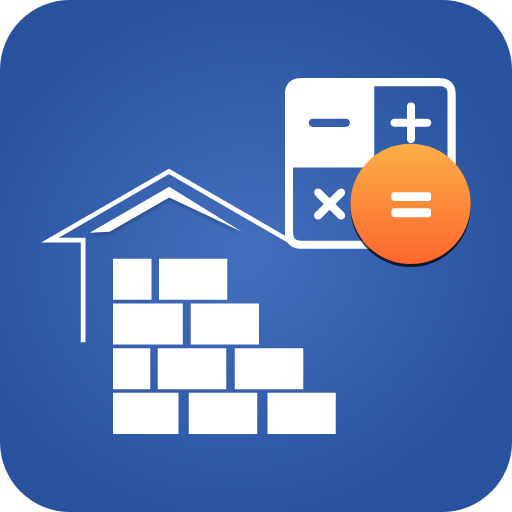# 13 Nov 2022 App Of The Day

## Construction Estimator

### by Hura technology

Free
236
Categories Tags
###### Description

Do you need a construction calculator to get an overall estimate for building a house?

The construction estimator app calculates the quantity of the construction material. You can estimate the quantity and cost of steel bars with a construction estimator. The construction calculator finds the number of paint buckets, cement bags for the ceiling, and wall plaster required for the building. You can calculate the number of blocks and bricks by putting construction data in the construction calculator for civil engineers. With this construction estimator calculator, you can also find the number of tiles required for construction. You can find the overall construction cost with our construction estimate and costing app. By using the excellent features of the residential construction estimator, you can get an estimate of individual materials as well. The building estimation becomes easy with a construction calculator for civil engineers.

How to use the construction calculator for civil engineers?

Construction estimate and costing app calculates the total material cost for construction. You can use this construction calculator to estimate the quantity of material and cost of a given construction area. The construction estimator calculator helps you calculate the overall cost of a house. With the construction estimate and costing app, you can get a construction estimate by providing the given construction area and your construction budget to this construction calculator. You can get a quick cost summary of the total construction in the construction estimator calculator.

What features do we provide in this construction estimate and costing app?

-Construction Estimator
Enter Built-up Area and Approx. Cost (Per Square Feet) in our construction calculator for civil engineers to calculate the overall quantity of material and overall cost of construction. You can save all the construction calculations by pressing the save work button in the construction estimator calculator. The material estimation feature of the construction material estimator app can make calculations of every single unit involved in construction.

-Measurement tools
You can get an easy way to level the building objects with bubble level in the construction material estimator app. You can measure the length of objects with the ruler of the construction estimator app. The construction estimator calculator also has a protractor that allows you to calculate the angle of objects from a specific surface.

-Bricks and blocks work
You can use the bricks calculator feature of the construction estimator calculator to estimate the total numbers of bricks, cement, and sand required for building a room. The construction estimator also works as a block calculator that allows you to calculate the quantity of material of blocks for a room construction.

Other features of the construction estimator app include:
• A plaster material calculator in the residential construction estimator estimates the quantity of cement and sand for a room construction.
• The floor tiles estimator in the construction calculator estimates the tiles and cement for rooms.
• The construction estimate and costing app calculates the quantity of concrete and steel required for stairs in a building.
• You can estimate paint for a whole building with this construction estimator calculator.
• Find cost of steel bars with the construction cost estimator app.
• A quick notepad in the construction material estimator app allows you to capture what is on your mind. You can also edit your notes in the residential construction estimator.

103